# Counting Rows of Even Numbers in Two Parallel Ranges

In this article, we are going to learn how to count rows of even numbers in two parallel ranges in Microsoft Excel.

To solve this problem, we will use SUMPRODUCT & MOD function.

• SUMPRODUCT function is used to count the number on the basis of criteria
• MOD function is used to check whether the number is even or odd

Let’s take an example and understand:-

We have numbers in two columns:Column A and Column B.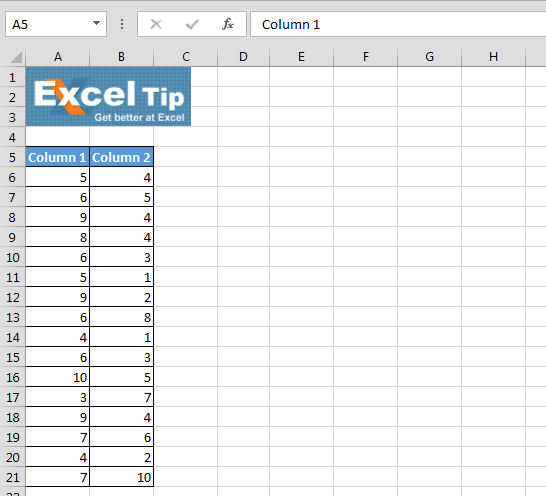• Enter the below formula in cell C6
• =SUMPRODUCT((MOD(\$A\$6:\$A\$21,2)=0)*(MOD(\$B\$6:\$B\$21,2)=0))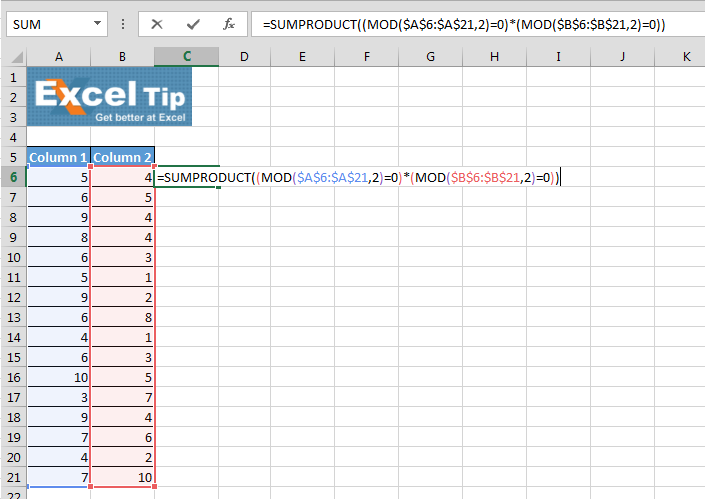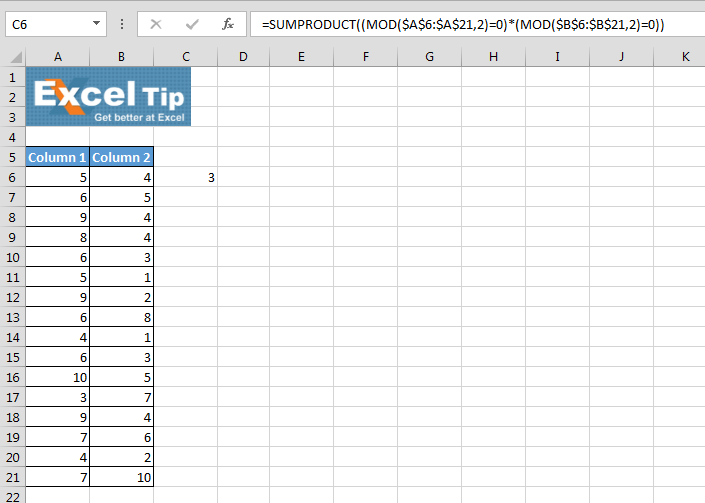In the above image showing data, you can see thatthere are 3 rows in which we have even numbers.

Let’s take another example:-

Let’s say we have same number in vertical position then how do we calculate the same calculation?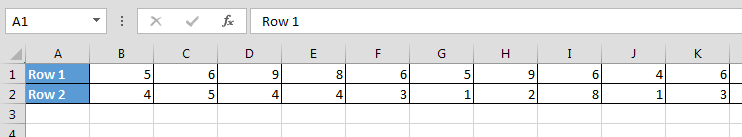• Enter the formula in cell B4
• =SUMPRODUCT((MOD(\$B\$1:\$Q\$1,2)=0)*(MOD(\$B\$2:\$Q\$2,2)=0))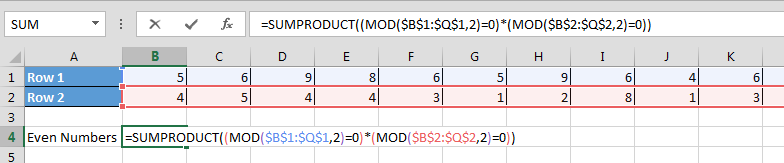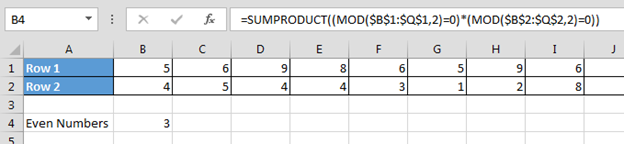Now you can see in the above image that we have just changed the cell reference, and we are getting accurate results.